We think you are located in Nigeria. Is this correct?

# Test yourself now

High marks in maths are the key to your success and future plans. Test yourself and learn more on Siyavula Practice.

# Chapter 7: Simple equations

In Chapter 6 you learnt that an algebraic expression is a mathematical phrase or statement. Algebraic expressions do not have equals signs.

In this chapter we will work with algebraic equations. Algebraic equations are mathematical sentences that do have equals signs. You will learn how to formulate and solve algebraic equations.

## 7.1 Comparing expressions and equations

You have learnt about the difference between algebraic expressions and algebraic equations. An algebraic equation is a mathematical sentence that contains a combination of numbers, symbols, variables and mathematical operators. It always has an equals sign.

algebraic equation An algebraic equation is a mathematical sentence that contains a combination of numbers, symbols, variables and mathematical operators. It always has an equals sign.

The table lists some of the differences between algebraic expressions and algebraic equations.

Expression Equation
An expression is a phrase. An equation is a sentence.
We simplify an expression. We solve an equation.
An expression does not have an equals sign. An equation has an equals sign.
An expression gives the relationship between variables. An equation shows that two expressions are equal.
The variables in an expression can have a whole range of values. The variables in an equation usually have specific values.
Example: Two more than a number, written as $x+2$ Example: Two more than a number is five, written as $x+2=5$

Let's look at an example to explore this further.

Suppose a shop owner sells maize at $$₦\,x$$ per cup. She also sells rice per cup, at double the price of the maize. On a specific day, she sold 50 cups of maize and 45 cups of rice.

• We can write an expression for her income for the day: $50\times x+45\times 2x$
• We can simplify the expression to: $140x$
• Her income for the day is therefore: $$₦\,140x$$
• Her income depends on the cost of a cup of maize, which can be any positive number.
• If we know that her income for the day was $$₦\,7,700$$, we can write an equation: $50\times x+45\times 2x = 7,700$
• We can simplify the equation to: $140x=7,700$
• The only value that makes this sentence true is $55$, because $140 \times 55 = 7,700$.
• Now we know that the price of a cup of maize is $&#8358;\,55$ and the price of a cup of rice is $2\times55= &#8358;\,110$.

## 7.2 Solving equations

### The solution of an equation

Look at the following algebraic equation:

It is easy to see that the only value for $x$ that will make this equation true is 2. This is because $3+2=5$. We say the number 2 is the solution of the equation and we write it as:

When the find the solution of an equation, we say we solve the equation.

solution The solution of an equation is the value of the variable that makes the equation true.

solve When we solve an equation, we find the value of the variable that makes the equation true.

To solve an equation, we must isolate the variable. If $x$ is the variable, we need to end up with the equation in the form:

### Representing an equation as a pair of scales

Last year you learnt that we can represent an algebraic equations as a pair of scales. The picture shows what a pair of scales looks like. These scales are balanced, because nothing has been placed on them.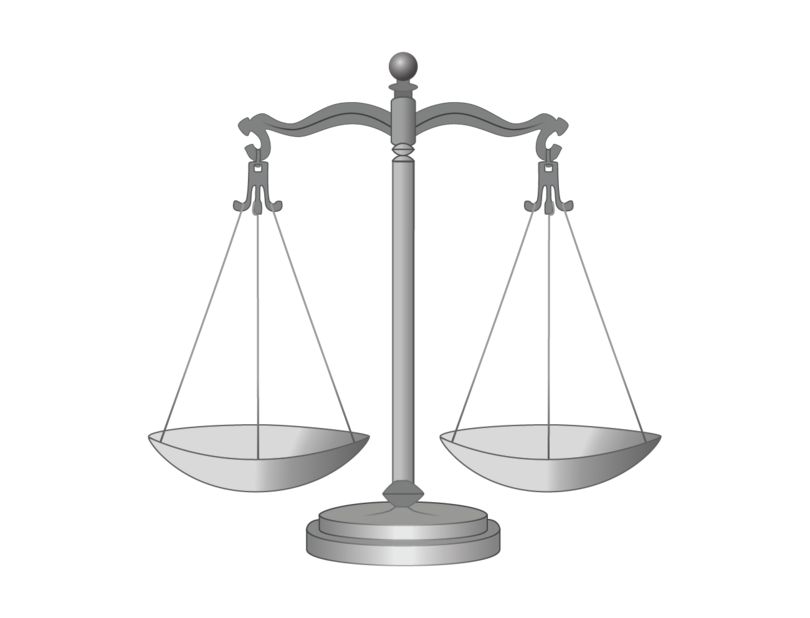If we place a mass piece on one of the scales, they become unbalanced.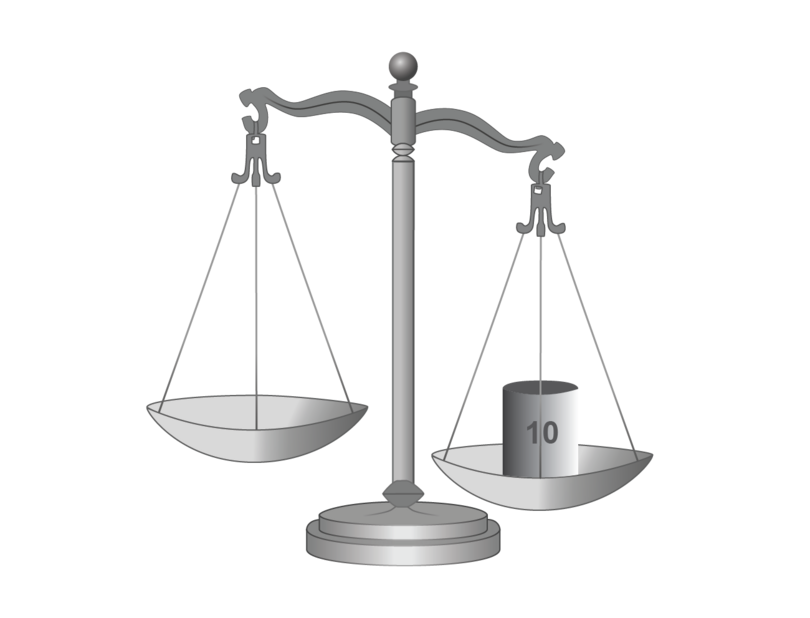We can balance the scales again by placing an identical mass piece on the other scale.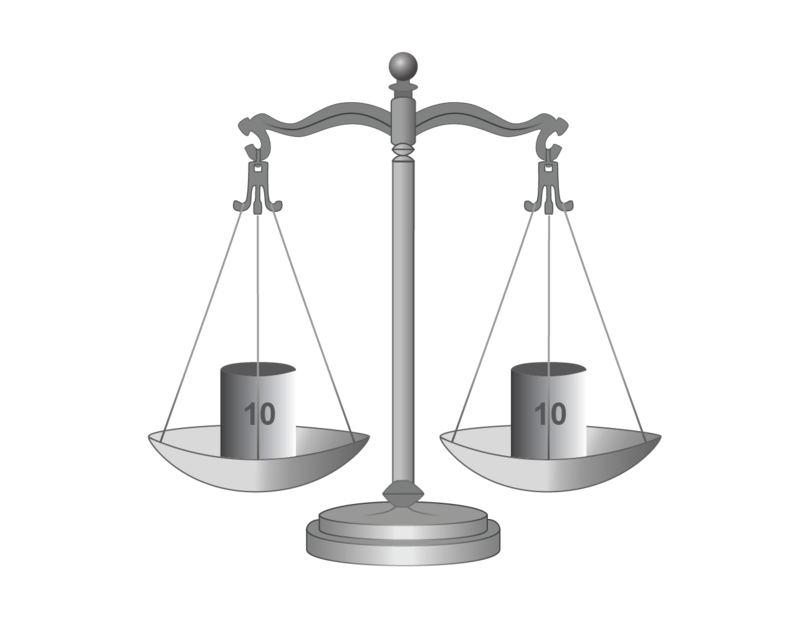As long as identical mass pieces are placed on both scales, the scales will remain in balance.

In an equation, the equals sign shows that two expressions (or an expression and a value) are equal to each other. We can think of each side of the equation as one of the scales above. As long as we do exactly the same on each side of the equation, the equation will remain true.

Consider the following algebraic equation:

We can represent it on a pair of scales as follows: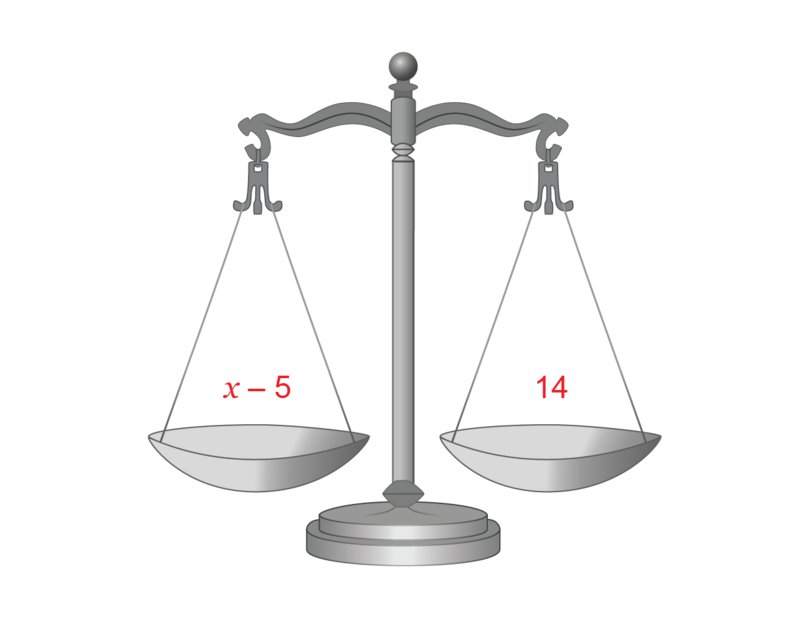Our aim is to isolate the variable. We must therefore "take away" the $-5$ from the left-hand scale. To do this, we add the additive inverse of $-5$.

Remember that addition and subtraction are inverse operations. A number and its additive inverse add up to zero, for example $8+(-8)=0$. Multiplication and division are inverse operations. When a number is multiplied by its multiplicative inverse, the answer is 1, for example $8\times\frac{1}{8}=1$

Whatever we add to the one scale, we must also add to the other scale. The scales now become: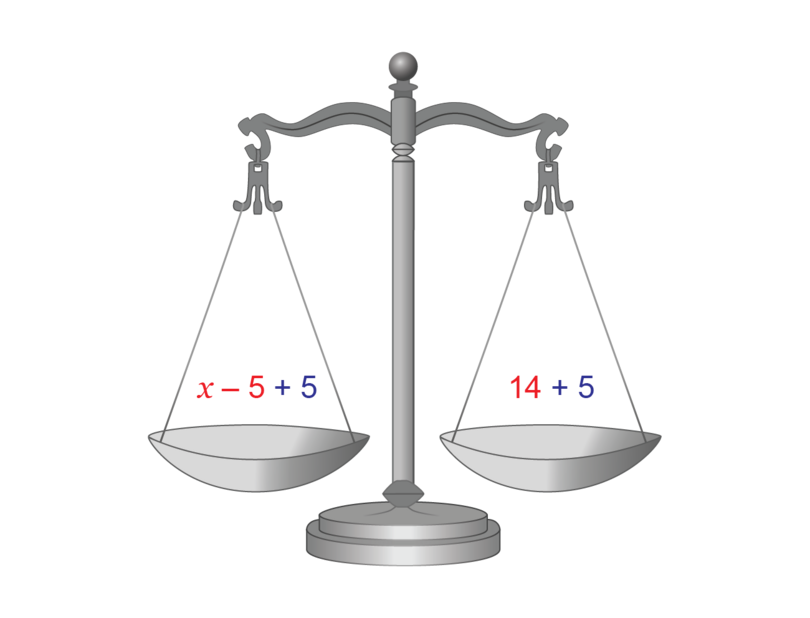If we simplify both sides, we get the solution of the equation:

### Solving simple equations and checking the solution

The following example from last year should refresh your memory on how to find the solution of a simple equation and then checking your solution.

### Worked example 7.1: Solving a simple equation and checking the solution

Solve the following equation and check the solution.

1. Step 1: If either side of the equation can be simplified, do that first.

We can add the like terms.

\begin{align} 2x+x-10&=2 \\ 3x-10&=2 \end{align}
2. Step 2: If there is a constant term on the same side as the variable, add its additive inverse. Remember to do this on both sides of the equation.

\begin{align} 2x+x-10&=2 \\ 3x-10\; \color{red}{+\;10}&=2\; \color{red}{+\;10} \\ 3x&=12 \end{align}
3. Step 3: If the variable has a coefficient, apply the multiplicative inverse of the coefficient. Remember to do this on both sides of the equation.

\begin{align} 2x+x-10 & = 2 \\ 3x-10 \; {\color{red}{+\;10}} & = 2 \; {\color{red}{+\;10}} \\ 3x & = 12 \\ {\color{orange}{\frac{1}{3} \times}} \frac{3x}{1} & = {\color{orange}{\frac{1}{3}\times}} \frac{12}{1} \\ \frac{3x}{3} & = \frac{12}{3} \\ \therefore x & = 4 \end{align}
4. Step 4: Check your solution. Substitute the solution into the left-hand side (LHS) of the original equation with the solution. Work out the value of the left-hand side.

Remember that to substitute means to replace the variable with the solution. In this case, you replace the $x$ with 4.

\begin{align} \text{LHS}&=2x+x-10 \\ &=2(4)+4-10 \\ &=8+4-10 \\ &=2 \end{align}

Remember that $2(4)$ means $2\times 4$.

5. Step 5: Repeat Step 4 for the right-hand side (RHS) of the original equation.

6. Step 6: Compare your answers from Steps 4 and 5. If they are the same, your solution is correct. If not, you made a mistake somewhere, and you need to solve the equation again.

$\text{LHS}=\text{RHS}$
The solution is correct.

### Exercise 7.1: Solve simple equations and check the solutions

Solve each of the following equations and check the solutions.

1. \begin{align} 4x+5-5&=17-5 \newline 4x&=12 \newline \frac{4x}{4}&=\frac{12}{4} \newline \therefore x&=3 \end{align}

Checking:

\begin{align} 4(3)+5&=17 \newline 12+5&=17 \newline 17&=17 \newline \therefore \text{LHS}&=\text{RHS} \end{align}
2. \begin{align} 13+3&=2x-3+3 \newline 16&=2x \newline \frac{16}{2}&=\frac{2x}{2} \newline 8&=x \newline \therefore x&=8 \end{align}

$8=x$ and $x=8$ has exactly the same meaning.

Checking:

\begin{align} 13&=2(8)-3 \newline 13&=16-3 \newline 13&=13 \newline \therefore \text{LHS}&=\text{RHS} \end{align}
3. \begin{align} \frac{1}{2}t+1-1&=5-1 \newline \frac{1}{2}t&=4 \newline \frac{2}{1}\times \frac{1}{2}t&=4\times \frac{2}{1} \newline \therefore t&=8 \end{align}

Checking:

\begin{align} \frac{1}{2}t+1&=5 \newline \frac{1}{2}(8)+1&=5 \newline \frac{1 \times 8}{2}+1&=5 \newline 4+1&=5 \newline 5&=5 \newline \therefore \text{LHS}&=\text{RHS} \end{align}
4. \begin{align} 36-36+\frac{3}{4}y&=44-36 \newline \frac{3}{4}y&=8 \newline \frac{4}{3}\times \frac{3}{4}y&=8\times \frac{4}{3} \newline y&=\frac{8\times4}{3} \newline \therefore y&=\frac{32}{3} \newline \end{align}

Checking:

\begin{align} 36+\frac{3}{4} \left(\frac{32}{3}\right) &=44 \newline 36+\frac{3\times 32}{4 \times3}&=44 \newline 36+\frac{3\times 32}{3 \times4}&=44 \newline 36+\frac{1\times 8}{1 \times1}&=44 \newline 36+8&=44 \newline 44&=44 \newline \therefore \text{LHS}&=\text{RHS} \end{align}
5. \begin{align} 1+9x&=19 \newline 1-1+9x&=19-1 \newline 9x&=18 \newline \frac{9x}{9}&=\frac{18}{9} \newline \therefore x&=2 \newline \end{align}

Checking:

\begin{align} 1-2(2)+11(2)&=19 \newline 1-4+22&=19 \newline 23-4&=19 \newline 19&=19 \newline \therefore \text{LHS}&=\text{RHS} \end{align}

Remember to always follow the correct order of operations. Use BODMAS: (Brackets, Of, Division, Multiplication, Addition, Subtraction).

6. \begin{align} 39&=21+7q-4q \newline 39&=21+3q \newline 39-21&=21-21+3q \newline 18&=3q \newline \frac{18}{3}&=\frac{3q}{3} \newline 6&=q \newline \therefore q&=6 \end{align}

Checking:

\begin{align} 39&=21+5(6)-4(6)+2(6) \newline 39&=21+30-24+12 \newline 39&=63-24 \newline 39&=39 \newline \therefore \text{LHS}&=\text{RHS} \end{align}
7. \begin{align} 8+4p-4p&=6p-4p-12 \newline 8&=2p-12 \newline 8+12&=2p-12+12 \newline 20&=2p \newline \frac{20}{2}&=\frac{2p}{2} \newline 10&=p \newline \therefore p&=10 \end{align}

Checking:

\begin{align} 8+4(10)&=6(10)-12 \newline 8+40&=60-12 \newline 48&=48 \newline \therefore \text{LHS}&=\text{RHS} \end{align}
8. \begin{align} 6a+7&=4a+13 \newline 6a-4a+7&=4a-4a+13 \newline 2a+7&=13 \newline 2a+7-7&=13-7 \newline 2a&=6 \newline \frac{2a}{2}&=\frac{6}{2} \newline \therefore a&=3 \end{align}

Checking:

\begin{align} 5(3)+7+3&=4(3)+13 \newline 15+7+3&=12+13 \newline 25&=25 \newline \therefore \text{LHS}&=\text{RHS} \end{align}
9. \begin{align} 6-3y+2y&=-2-2y+2y \newline 6-y&=-2 \newline 6-y+y&=-2+y \newline 6&=-2+y \newline 6+2&=-2+2+y \newline 8&=y \newline \therefore y&=8 \end{align}

You always need to have a positive variable as your final answer. If you end up with a negative variable, such as $-y$, simply add the positive of the variable on both sides of the equation.

Checking:

\begin{align} 6-3(8)&=-2-2(8) \newline 6-24&=-2-16 \newline -18&=-18 \newline \therefore \text{LHS}&=\text{RHS} \end{align}
10. \begin{align} 15-6t&=9t-30 \newline 15-6t-9t&=9t-9t-30 \newline 15-15t&=-30 \newline 15-15t+15t&=-30+15t \newline 15&=-30+15t \newline 15+30&=-30+30+15t \newline 45&=15t \newline \frac{45}{15}&=\frac{15t}{15} \newline 3&=t\newline \therefore t&=3 \end{align}

If you end up with a variable that has a negative coefficient, such as $-15t$, simply add the positive of the term on both sides of the equation.

Checking:

\begin{align} 10-6(3)+5&=5(3)-30+3+3(3) \newline 10-18+5&=15-30+3+9 \newline 15-18&=27-30 \newline -3&=-3 \newline \therefore \text{LHS}&=\text{RHS} \end{align}

Remember to always follow the correct order of operations by using BODMAS: (Brackets, Of, Division, Multiplication, Addition, Subtraction).

### Solving simple equations that have brackets

In Chapter 6 you learnt how to simplify algebraic expressions with brackets. You can apply this knowledge when you are solving algebraic equations with brackets.

### Worked example 7.2: Solving a simple equation with brackets and checking the solution

Solve the following equation and check the solution:

1. Step 1: Expand the brackets using the distributive law. Then, if there are like terms on either side of the equation, collect them.

\begin{align} 2(x+4)+x&=-2(x+1)+20 \\ 2x+8+x&=-2x-2+20 \\ 3x+8&=-2x+18 \end{align}

If necessary, go back to Chapter 6 and revise how to expand brackets.

2. Step 2: Use additive inverses to arrange the equation so that all the terms with variables are on the left-hand side and all the constant terms are on the right-hand side.

Remember to do exactly the same on both sides of the equation.

\begin{align} 2(x+4)+x&=-2(x+1)+20 \\ 2x+8+x&=-2x-2+20 \\ 3x+8&=-2x+18 \\ 3x+8{\color{red}{\;-\;8}}{\color{green}{\;+\;2x}}&=-2x{\color{green}{\;+\;2x}}+18 {\color{red}{\;-\;8}} \\ \end{align}
3. Step 3: Simplify both sides of the equation.

\begin{align} 2(x+4)+x&=-2(x+1)+20 \\ 2x+8+x&=-2x-2+20 \\ 3x+8&=-2x+18 \\ 3x+8{\color{red}{\;-\;8}}{\color{green}{\;+\;2x}}&=-2x{\color{green}{\;+\;2x}}+18 {\color{red}{\;-\;8}} \\ 3x+2x &=10 \\ 5x &=10 \end{align}
4. Step 4: If the variable has a coefficient, apply the multiplicative inverse of the coefficient. Remember to do this on both sides of the equation.

\begin{align} 2(x+4)+x&=-2(x+1)+20 \\ 2x+8+x&=-2x-2+20 \\ 3x+8&=-2x+18 \\ 3x+8{\color{red}{\;-\;8}}{\color{green}{\;+\;2x}}&=-2x{\color{green}{\;+\;2x}}+18 {\color{red}{\;-\;8}} \\ 3x+2x &=10 \\ 5x &=10 \\ {\color{orange}{\frac{1}{5}\times}} \frac{5x}{1}&={\color{orange}{\frac{1}{5}\times}}\frac{10}{1} \\ \end{align}
5. Step 5: Simplify both sides of the equation.

\begin{align} 2(x+4)+x&=-2(x+1)+20 \\ 2x+8+x&=-2x-2+20 \\ 3x+8&=-2x+18 \\ 3x+8{\color{red}{\;-\;8}}{\color{green}{\;+\;2x}}&=-2x{\color{green}{\;+\;2x}}+18 {\color{red}{\;-\;8}} \\ 3x+2x &=10 \\ 5x &=10 \\ {\color{orange}{\frac{1}{5}\times}} \frac{5x}{1}&={\color{orange}{\frac{1}{5}\times}} \frac{10}{1} \\ \frac{5x}{5}&=\frac{10}{5} \\ \therefore x&=2 \end{align}
6. Step 6: Check your solution. Replace the variable on the left-hand side (LHS) of the original equation with the solution. Work out the value of the left-hand side.

\begin{align} \text{LHS}&=2(x+4)+x \\ &=2(2+4)+2 \\ &=2(6)+2 \\ &=12+2\\ &=14 \end{align}
7. Step 7: Repeat Step 6 for the right-hand side (RHS) of the original equation.

\begin{align} \text{RHS}&=-2(x+1)+20 \\ &=-2(2+1)+20 \\ &=-2(3)+20 \\ &=-6+20\\ &=14 \end{align}
8. Step 8: Compare your answers from Steps 6 and 7. If they are the same, your solution is correct. If not, you made a mistake somewhere. Solve the equation again.

$\text{LHS}=\text{RHS}$
The solution is correct.

### Exercise 7.2: Solve simple equations with brackets and check the solutions

Solve each of the following equations and check the solutions.

1. \begin{align} 2(y+3)&=10 \\ 2y+6&=10 \\ 2y+6-6&=10-6 \\ 2y&=4 \\ \frac{2y}{2}&=\frac{4}{2} \\ \therefore y&=2 \end{align}

Checking:

\begin{align} 2(2+3)&=10 \\ 2(5)&=10 \\ 10&=10 \\ \therefore \text{LHS}&=\text{RHS} \end{align}
2. \begin{align} 4(a-2)&=a+1 \\ 4a-8&=a+1 \\ 4a-8+8-a &= a-a+1+8 \\ 3a&=9 \\ \frac{3a}{3}&=\frac{9}{3} \\ \therefore a&=3 \end{align}

Checking:

\begin{align} 4(a-2)&=a+1 \\ 4(3-2)&=3+1 \\ 4(1)&=4 \\ 4&=4 \\ \therefore \text{LHS}&=\text{RHS} \end{align}
3. \begin{align} 3(x+4)&=8-x \\ 3x+12&=8-x \\ 3x+12-12+x &= 8-x+x-12 \\ 4x&=-4\\ \frac{4x}{4}&=\frac{-4}{4} \\ \therefore x&=-1 \end{align}

Checking:

\begin{align} 3(x+4)&=8-x \\ 3(-1+4)&=8-(-1) \\ 3(3)&=8+1 \\ 9&=9 \\ \therefore \text{LHS}&=\text{RHS} \end{align}
4. \begin{align} 2(y+1)&=6(y-1) \\ 2y+2&=6y-6 \\ 2y+2-2-6y &= 6y-6y-6-2 \\ -4y&=-8\\ \frac{-4y}{-4}&=\frac{-8}{-4} \\ \therefore y&=2 \end{align}

Checking:

\begin{align} 2(y+1)&=6(y-1) \\ 2(2+1)&=6(2-1) \\ 2(3)&=6(1) \\ 6&=6 \\ \therefore \text{LHS}&=\text{RHS} \end{align}
5. \begin{align} 4(t+2)-3(2t-1)&=-1 \\ 4t+8-6t+3&=-1 \\ -2t+11 &= -1 \\ -2t+11-11&=-1-11\\ -2t &= -12 \\ \frac{-2t}{-2}&=\frac{-12}{-2} \\ \therefore t&=6 \end{align}

Checking:

\begin{align} 4(t+2)-3(2t-1)&=-1 \\ 4(6+2)-3(2(6)-1)&=-1 \\ 4(8)-3(11)&=-1\\ 32-33&=-1 \\ -1&=-1 \\ \therefore \text{LHS}&=\text{RHS} \end{align}
6. \begin{align} 3(x-3)+3(2+x)&=0 \\ 3x-9+6+3x&=0 \\ 6x-3 &= 0 \\ 6x-3+3&=0+3\\ 6x &= 3 \\ \frac{6x}{6}&=\frac{3}{6} \\ \therefore x&=\frac{1}{2} \end{align}

Checking:

\begin{align} 3(x-3)+3(2+x)&=0 \\ 3(\frac{1}{2}-3)+3(2+\frac{1}{2})&=0 \\ 3(-2\frac{1}{2})+3(2\frac{1}{2})&=0\\ \frac{3}{1}\times\frac{-5}{2}+\frac{3}{1}\times\frac{5}{2}&=0 \\ \frac{-15}{2}+\frac{15}{2}&=0 \\ 0&=0 \\ \therefore \text{LHS}&=\text{RHS} \end{align}

Remember to always follow the correct order of operations using BODMAS: (Brackets, Of, Division, Multiplication, Addition, Subtraction).

7. \begin{align} 5(p-1)-3(2p+1)&=0 \\ 5p-5-6p-3&=0 \\ -p-8 &= 0 \\ -p-8+8&=0+8\\ -p &= 8 \\ \frac{-p}{-1}&=\frac{8}{-1} \\ \therefore p&=-8 \end{align}

Checking:

\begin{align} 5(p-1)-3(2p+1)&=0 \\ 5(-8-1)-3(2(-8)+1)&=0 \\ 5(-9)-3(-16+1)&=0\\ -45-3(-15)&=0 \\ -45+45&=0 \\ 0&=0 \\ \therefore \text{LHS}&=\text{RHS} \end{align}
8. \begin{align} 19(b-1)+8&=-(b+1) \\ 19b-19+8&=-b-1 \\ 19b-11 &= -b-1 \\ 19b-11+11+b&=-b+b-1+11\\ 20b &= 10 \\ \frac{20b}{20}&=\frac{10}{20} \\ b&=\frac{1}{2} \end{align}

Checking:

\begin{align} 19(b-1)+8&=-(b+1) \\ 19(\frac{1}{2}-1)+8&=-(\frac{1}{2}+1) \\ 19(-\frac{1}{2})+8&=-(1\frac{1}{2})\\ \frac{19}{1}\times\frac{-1}{2}+\frac{8}{1}&=-1\frac{1}{2} \\ \frac{-19}{2}+\frac{16}{2}&=\frac{-3}{2} \\ \frac{-3}{2}&=\frac{-3}{2} \\ \therefore \text{LHS}&=\text{RHS} \end{align}

Remember that when you are adding or subtracting fractions, first find equivalent fractions that all have the same denominator. Then add or subtract the numerators.

9. \begin{align} 2(5a-3)-5(7a-3)&=2a \\ 10a-6-35a+15&=2a \\ -25a+9 &= 2a \\ -25a+9-9-2a&=2a-2a-9\\ -27a &= -9 \\ \frac{-27a}{-27}&=\frac{-9}{-27} \\ \therefore a&=\frac{1}{3} \end{align}

Checking:

\begin{align} 2(5a-3)-5(7a-3)&=2a \\ 2(\frac{5}{1} \times \frac{1}{3}-3) - 5(\frac{7}{1} \times\frac{1}{3}-3)&=\frac{2}{1}\times\frac{1}{3} \\ 2(\frac{5}{3}-\frac{9}{3})-5(\frac{7}{3}-\frac{9}{3})&=\frac{2}{3}\\ \frac{2}{1}(\frac{-4}{3})-\frac{5}{1}(\frac{-2}{3})&=\frac{2}{3}\\ \frac{-8}{3}+\frac{10}{3}&=\frac{2}{3}\\ \frac{2}{3}&=\frac{2}{3} \\ \therefore \text{LHS}&=\text{RHS} \end{align}
10. In Chapter 6 you learnt the following:

• $(a+b)^2=a^2+2ab+b^2$
• $(a+b)(a-b)=a^2-b^2$
\begin{align} (2x-3)^2&=4(x-1)(x+1)+1 \\ 4x^2-12x+9&=4(x^2-1)+1 \\ 4x^2-12x+9&=4x^2-4+1 \\ 4x^2-12x+9&=4x^2-3 \\ 4x^2-4x^2-12x+9-9 &= 4x^2-4x^2-3-9 \\ -12x&=-12\\ \frac{-12x}{-12}&=\frac{-12}{-12} \\ \therefore x&=1 \end{align}

Checking:

\begin{align} (2x-3)^2&=4(x-1)(x+1)+1 \\ (2(1)-3)^2&=4(1-1)(1+1)+1 \\ (2-3)^2&=4(0)(2)+1\\ (-1)\times(-1)&=0+1 \\ 1&=1 \\ \therefore \text{LHS}&=\text{RHS} \end{align}

Remember, if you multiply any number or variable by zero, the answer is zero.

### Solving simple equations that have fractions

In Chapter 6 you learnt how to simplify algebraic expressions with fractions. You can apply this knowledge to solve algebraic equations with fractions.

### Worked example 7.3: Solving a simple equation with fractions and checking the solution

Solve the following equation and check the solution:

1. Step 1: Find the LCD of the denominators.

The LCD of $3$ and $6x$ is $6x$.

If necessary, go back to Chapter 6 and revise how to find the LCD of denominators that contain variables.

2. Step 2: Multiply all the terms of the equation with the LCD from Step 1.

Note that we are doing exactly the same on both sides of the equation.

\begin{align} {\color{red}{\frac{6x}{1}\times}} \; \frac{2x-1}{3} & = \frac{4x^2-2}{6x} {\color{red}{\times\frac{6x}{1}}}\\ \end{align}
3. Step 3: For each term of the equation, find the HCF of the denominator and the LCD from Step 1. Divide by the HCF.

In the term on the left side of the equation, the HCF of $6x$ and $3$ is $3$. Therefore, $6x \div 3=2x$ and $3 \div 3=1$.

In the term on the right side of the equation, the HCF of $6x$ and $6x$ is $6x$. And $6x \div 6x=1$.

\begin{align} \require{cancel} {\color{red}{\frac{6x}{1}\times}} \; \frac{2x-1}{3} & = \frac{4x^2-2}{6x} {\color{red}{\times\frac{6x}{1}}}\\ \frac{\color{green}{\cancel{6x}^{2x}}}{1} \times \frac{2x-1}{\color{green}{\cancel{3}^1}} &=\frac{4x^2-2}{\color{green}{\cancel{6x}^1}} \times \frac{\color{green}{\cancel{6x}^1}}{1}\\ \end{align}
4. Step 4: Multiply the factors that remain in each term.

\begin{align} {\color{red}{\frac{6x}{1}\times}} \; \frac{2x-1}{3}&=\frac{4x^2-2}{6x}{\color{red}{\times\frac{6x}{1}}}\\ \frac{\color{green}{\cancel{6x}^{2x}}}{1} \times \frac{2x-1}{\color{green}{\cancel{3}^1}} &=\frac{4x^2-2}{\color{green}{\cancel{6x}^1}} \times \frac{\color{green}{\cancel{6x}^1}}{1}\\ 2x(2x-1)&=4x^2-2 \end{align}

Remember that a number or variable remains the same if you divide or multiply it by 1, for example $2x\times 1=2x$ and $\frac{5x}{1}=5x$.

5. Step 5: Solve the equation as you did in the previous section.

\begin{align} 2x(2x-1)&=4x^2-2 \\ 4x^2-2x&=4x^2-2 \\ 4x^2-4x^2-2x&=4x^2-4x^2-2 \\ -2x&=-2 \\ \frac{-2x}{-2}&-\frac{-2}{-2} \\ \therefore x&=1 \end{align}
6. Step 6: Check your solution. Remember to substitute the solution for the variable in the original equation.

\begin{align} \frac{2x-1}{3}&=\frac{4x^2-2}{6x} \\ \frac{2(1)-1}{3}&=\frac{4(1)^2-2}{6(1)} \\ \frac{2-1}{3}&=\frac{4(1)(1)-2}{6(1)}\\ \frac{1}{3}&=\frac{2}{6} \\ \frac{1}{3}&=\frac{1}{3} \\ \therefore \text{LHS}&=\text{RHS} \end{align}

### Exercise 7.3: Solve simple equations with fractions and check the solutions

Solve each of the following equations and check the solutions.

1. LCD of denominators is $3x$, so multiply both sides by $\frac{3x}{1}$. Then divide by the HCFs on each side of the equation.

\begin{align} \frac{3x}{1}\times\frac{2}{x}&=\frac{1}{3} \times \frac{3x}{1} \\ \frac{\cancel{3x}^3}{1}\times\frac{2}{\cancel x^1}&=\frac{1}{\cancel 3^1} \times \frac{\cancel{3x}^x}{1}\\ 6&=x \\ \therefore x&=6 \\ \end{align}

Checking:

\begin{align} \frac{2}{6}&=\frac{1}{3} \\ \frac{1}{3}&=\frac{1}{3} \\ \therefore \text{LHS}&=\text{RHS} \end{align}
2. LCD of denominators is $6a$. The HCF on the left side is $6a$, and on the right side is $3$.

\begin{align} \frac{6a}{1}\times\frac{8}{6a}&=\frac{2}{3} \times \frac{6a}{1} \\ \frac{\cancel{6a}^1}{1}\times\frac{8}{\cancel {6a}^1}&=\frac{2}{\cancel 3^1} \times \frac{\cancel{6a}^{2a}}{1}\\ 8&=4a \\ \frac{4a}{4}&=\frac{8}{4} \\ \therefore a&=2 \end{align}

Checking:

\begin{align} \frac{8}{6(2)}&=\frac{2}{3} \\ \frac{8}{12}&=\frac{2}{3} \\ \frac{2}{3}&=\frac{2}{3} \\ \therefore \text{LHS}&=\text{RHS} \end{align}
3. Remember that you need to multiply each term by the LCD.

Also, you can only divide by a HCF within a term. So when there is a $+$ or $-$ sign between terms, you cannot divide by the HCF.

\begin{align} 1+\frac{p}{4}&=\frac{p}{5} \\ \frac{20}{1}\times 1+\frac{20}{1}\times \frac{p}{4}&=\frac{p}{5}\times \frac{20}{1} \\ \frac{20}{1}+\frac{\cancel{20}^5}{1}\times \frac{p}{\cancel 4^1}&=\frac{p}{\cancel 5^1}\times \frac{\cancel{20}^4}{1} \\ 20+5p&=4p\\ 20-20+5p-4p&=4p-4p-20 \\ \therefore p&=-20 \end{align}

Checking:

\begin{align} 1+\frac{-20}{4}&=\frac{-20}{5} \\ 1-5&=-4 \\ -4&=-4 \\ \therefore \text{LHS}&=\text{RHS} \end{align}
4. \begin{align} \frac{2y}{1}\times\frac{3}{2}-\frac{2y}{1}\times\frac{4}{y}&=1\times\frac{2y}{1} \\ \frac{\cancel{2y}^y}{1}\times\frac{3}{\cancel 2^1}-\frac{\cancel{2y}^2}{1}\times\frac{4}{\cancel y^1}&=\frac{2y}{1} \\ 3y-8 &= 2y \\ 3y-2y-8+8&=2y-2y+8\\ \therefore y&=8 \\ \end{align}

Checking:

\begin{align} \frac{3}{2}-\frac{4}{8}&=1 \\ \frac{3}{2}-\frac{1}{2}&=1 \\ \frac{2}{2}&=1 \\ 1&=1 \\ \therefore \text{LHS}&=\text{RHS} \end{align}
5. \begin{align} \frac{2n-1}{4}&=0 \\ \frac{4}{1} \times \frac{2n-1}{4}&=0 \times \frac{4}{1} \\ \frac{\cancel 4^1}{1} \times \frac{2n-1}{\cancel 4^1}&=0 \times \frac{4}{1} \\ 2n-1&=0\\ 2n-1+1&=0+1 \\ 2n&=1 \\ \frac{2n}{2}&=\frac{1}{2} \\ \therefore n&=\frac{1}{2} \end{align}

Checking:

\begin{align} \frac{\frac{2}{1}(\frac{1}{2})-1}{4}&=0 \\ \frac{1-1}{4}&=0 \\ \frac{0}{4}&=0\\ 0&=0 \\ \therefore \text{LHS}&=\text{RHS} \end{align}
6. \begin{align} \frac{x-1}{15}&=\frac{1}{3} \\ \frac{15}{1}\times\frac{x-1}{15}&=\frac{1}{3}\times\frac{15}{1} \\ \frac{\cancel{15}^1}{1}\times\frac{x-1}{\cancel{15}^1}&=\frac{1}{\cancel 3^1}\times\frac{\cancel{15}^5}{1} \\ x-1&=5\\ x-1+1&=5+1 \\ \therefore x&=6 \end{align}

Checking:

\begin{align} \frac{6-1}{15}&=\frac{1}{3} \\ \frac{5}{15}&=\frac{1}{3} \\ \frac{1}{3}&=\frac{1}{3}\\ \therefore \text{LHS}&=\text{RHS} \end{align}
7. \begin{align} \frac{b-1}{2}&=\frac{b+1}{4} \\ \frac{4}{1}\times\frac{b-1}{2}&=\frac{b+1}{4}\times\frac{4}{1} \\ \frac{\cancel 4^2}{1}\times\frac{b-1}{\cancel 2^1}&=\frac{b+1}{\cancel 4^1}\times\frac{\cancel 4^1}{1} \\ 2(b-1)&=b+1\\ 2b-2&=b+1 \\ 2b-b-2+2&=b-b+1+2\\ \therefore b&=3 \\ \end{align}

Checking:

\begin{align} \frac{3-1}{2}&=\frac{3+1}{4} \\ \frac{2}{2}&=\frac{4}{4} \\ 1&=1 \\ \therefore \text{LHS}&=\text{RHS} \end{align}
8. \begin{align} \frac{c+2}{3}&=\frac{c-2}{1} \\ \frac{3}{1}\times\frac{c+2}{3}&=\frac{c-2}{1}\times\frac{3}{1} \\ \frac{\cancel 3^1}{1}\times\frac{c+2}{\cancel 3^1}&=\frac{c-2}{1}\times\frac{3}{1} \\ c+2&=3(c-2)\\ c+2&=3c-6 \\ c-3c+2-2&=3c-3c-6-2\\ -2c&=-8 \\ \frac{-2c}{-2}&=\frac{-8}{-2}\\ \therefore c&=4 \end{align}

Checking:

\begin{align} \frac{4+2}{3}&=4-2 \\ \frac{6}{3}&=2 \\ 2&=2 \\ \therefore \text{LHS}&=\text{RHS} \end{align}
9. \begin{align} \frac{x-1}{3}+\frac{2-x}{2}&=1 \\ \frac{6}{1}\times\frac{x-1}{3}+\frac{6}{1}\times\frac{2-x}{2}&=1 \times \frac{6}{1} \\ \frac{\cancel 6^2}{1}\times\frac{x-1}{\cancel 3^1}+\frac{\cancel 6^3}{1}\times\frac{2-x}{\cancel 2^1}&=\frac{6}{1} \\ 2(x-1)+3(2-x)&=6 \\ 2x-2+6-3x&=6\\ -x+4&=6\\ -x+4-4&=6-4\\ -x&=2\\ \frac{-x}{-1}&=\frac{2}{-1} \\ \therefore x&=-2 \end{align}

Checking:

\begin{align} \frac{-2-1}{3}+\frac{2-(-2)}{2}&=1 \\ \frac{-3}{3}+\frac{4}{2}&=1 \\ -1+2&=1 \\ 1&=1 \\ \therefore \text{LHS}&=\text{RHS} \end{align}
10. \begin{align} \frac{2y-1}{4}&=\frac{y^2+1}{2y}\\ \frac{4y}{1}\times\frac{2y-1}{4}&=\frac{y^2+1}{2y}\times\frac{4y}{1} \\ \frac{\cancel{4y}^y}{1}\times\frac{2y-1}{\cancel 4^1}&=\frac{y^2+1}{\cancel{2y}^1}\times\frac{\cancel{4y}^2}{1} \\ y(2y-1)&=2(y^2+1) \\ 2y^2-y&=2y^2+2 \\ 2y^2-2y^2-y&=2y^2-2y^2+2 \\ -y&=2\\ \frac{-y}{-1}&=\frac{2}{-1} \\ \therefore y&=-2 \end{align}

Checking:

\begin{align} \frac{2(-2)-1}{4}&=\frac{(-2)^2+1}{2(-2)}\\ \frac{-4-1}{4}&=\frac{(-2)(-2)+1}{2(-2)}\\ \frac{-5}{4}&=\frac{4+1}{-4}\\ \frac{-5}{4}&=\frac{5}{-4} \\ -\frac{5}{4}&=-\frac{5}{4} \\ \therefore \text{LHS}&=\text{RHS} \end{align}

## 7.3 Using equations to solve problems

Equations are extremely useful when you need to solve problems that are described in words. Last year you learnt that these steps can be used to solve a problem described in words:

1. Understand the problem. Read the question carefully a few times. Identify the information you have. Make sure you know what is asked. It may help to draw a picture.
2. Make a plan. Identify the unknown(s). Make one unknown $x$. If there is more than one unknown, express all of them in terms of $x$. Write an equation that you can use to solve the problem.
3. Carry out the plan. Solve the equation.
4. Look back. Check your solution(s). Make sure that you give the answers to everything that was asked.

In Chapter 6 you were given some examples of ways to write word phrases as mathematical statements. Revise this before you proceed.

### Worked example 7.4: Solving a problem using an equation

The length of a rectangle with a perimeter of 120 cm is 10 cm longer than its breadth. Determine the length and the breadth of the rectangle in centimetres.

1. Step 1: Understand the problem.

• Given: $P =$ 120 cm
• Given: Length equals breadth plus 10 cm
2. Step 2: Devise a plan.

• Breadth: $x$
• Length: $x+10$
• For a rectangle: $P=2l+2b$

The equation is:

3. Step 3: Carry out the plan.

\begin{align} 120 &= 2(x+10) + 2x \newline 120 &= 2x + 20 +2x \newline 120 &= 4x + 20 \newline 120-20 &= 4x+20-20 \newline 100&=4x \newline \frac{100}{4}&=\frac{4x}{4} \newline \therefore 25&=x \end{align}

Therefore:

\begin{align} \text{Breadth} &= 25\text{ cm} \newline \text{Length}\phantom{0} &= 25+10 \newline &=35\text{ cm} \newline \end{align}
4. Step 4: Look back.

\begin{align} 120 &= 2(x+10) + 2x \newline 120 &= 2(25+10) +2(25) \newline 120&=2(35)+50 \newline 120&=70+50 \newline 120&=120 \newline \therefore \text{LHS}&=\text{RHS} \end{align}

The length is 35 cm and the breadth is 25 cm.

### Exercise 7.4: Use equations to solve problems

1. If a number is doubled and 10 is then added to it, the answer is 60. Determine the number.

Let the number be $x$.

\begin{align} 2x+10&=60 \\ 2x+10-10&=60-10 \\ 2x&=50 \\ \frac{2x}{2}&=\frac{50}{2}\\ \therefore x&=25 \end{align}

The number is 25.

2. A mother is three times as old as her daughter. In 15 years' time, their combined ages will be 78 years. Determine their current ages.

Let the daughter's current age be $x$. In 15 years, her age will be $x+15$.
The mother's current age is $3x$. In 15 years, her age will be $3x+15$.

\begin{align} x+15+3x+15&=78 \\ 4x+30&=78 \\ 4x+30-30&=78-30 \\ 4x&=48 \\ \frac{4x}{4}&=\frac{48}{4}\\ \therefore x&=12 \end{align}

The daughter is currently 12 years old.
The mother is currently $12\times3$ = 36 years old.

3. The second side of a triangle is twice is long as the first side. The third side is 1 cm longer than the first side. If the perimeter of the triangle is 21 cm, determine length of each side.

Let the first side be $x$.
The second side is $2x$.
The third side is $x+1$.

\begin{align} x+2x+x+1&=21 \\ 4x+1&=21 \\ 4x+1-1&=21-1 \\ 4x&=20 \\ \frac{4x}{4}&= \frac{20}{4} \\ \therefore x &= 5 \end{align}

The first side is 5 cm.
The second side is $2 \times 5$ = 10 cm.
The third side is $5+1$ = 6 cm.

4. The length of a rectangular schoolground is 700 m longer than its breadth. The perimeter of the schoolground is 6,200 m. Determine the length and breadth of the schoolground.

Let the breadth be $x$.
Then the length is $x+700$.

Remember, the perimeter of a rectangle is given by $P=2l+2b$.

\begin{align} P&=2l+2b \\ 6,200&=2(x+700)+2x \\ 6,200&=2x+1,400+2x \\ 6,200&=1,400+4x \\ 6,200-1,400&=1,400-1,400+4x \\ 4,800&=4x\\ \frac{4,800}{4} &= \frac{4x}{4} \\ \therefore 1,200 &= x \end{align}

The breadth of the schoolground is 1,200 m, and the length is $1,200+700$ = 1,900 m.

5. The difference in the area of two squares is 21 square units. The side length of the smaller square is 1 unit shorter than the side length of the larger square. Determine the side length of each square.

Let the side length of the larger square be $x$.
Then the side length of the smaller square is $x-1$.

Remember, the area of a square is given by $A=s^2$.

\begin{align} A_\text{larger}-A_\text{smaller}&= x^2-(x-1)^2 \\ 21&=x^2-(x^2-2x+1) \\ 21&=x^2-x^2+2x-1 \\ 21&=2x-1 \\ 21+1&=2x-1+1 \\ 22&=2x \\ \frac{2x}{2}&=\frac{22}{2} \\ \therefore x&=11 \newline \end{align}

The larger square has a side length of 11 units.
The smaller square has a side length of $11-1$ = 10 units.

6. Lami has 30 sweets and Chinweike has 14 sweets. How many sweets must Chinweike give Lami for Lami to have three times as many sweets as Chinweike?

Let the number of sweets that Chinweike gives Lami be $x$.
Then Lami will have $30+x$ sweets and Chinweike will have $14-x$ sweets.
Lami must end up with three times as many sweets, so three times what Chinweike has, which is $3(14-x)$, must be equal to what Lami has, which is $30+x$.

\begin{align} 3(14-x)&=30+x \\ 42-3x&=30+x \\ 42-42-3x-x&=30+x-x-42 \\ -4x&=-12 \\ \frac{-4x}{-4}&=\frac{-12}{-4} \\ \therefore x&=3 \newline \end{align}

Chinweike must give Lami 3 sweets.

7. A car travels 150 km in 90 minutes. Determine the average speed of the car in km/h.

Remember that you can use the formula $\text{speed}=\frac{\text{distance}}{\text{time}}$ to work out speed.

You are asked to give the speed in kilometres per hour, so you need to convert 90 minutes to hours. To do this, you divide by 60.

Let the average speed be $x\text{ km/h}$.

\begin{align} x &=\frac{150}{\frac{90}{60}} \\ &= \frac{150}{1}\times\frac{60}{90} \\ &=\frac{150}{1}\times\frac{\cancel{60}^2}{\cancel{90}^3} \\ &= \frac{150}{1}\times\frac{2}{3} \\ &= \frac{300}{3} \\ &=100 \\ \end{align}

The average speed of the car is 100 km/h.

8. Latifah can walk in a straight line from her house to the shop. She walks straight towards the shop. She walks 20% of the distance and then realises that she has forgotten her purse at home. She turns around and walks straight back to her house again. When she has walked 2% of the distance between her house and the shop, her cell phone rings and she stops to answer it. At this point, she is 9 m away from her house. Determine the distance between her house and the shop.

Let the distance be $x$.
She walks $20\%\times x$ towards the shop and then $2\% \times x$ back.

\begin{align} (20\%\times x)-(2\%\times x) &=9 \\ \frac{20}{100}\times \frac{x}{1}-\frac{2}{100}\times \frac{x}{1} &= 9\\ \frac{20x}{100}-\frac{2x}{100} &= \frac{900}{100}\\ \frac{18x}{100} &=\frac{900}{100} \\ 18x&=900 \\ \frac{18x}{18}&=\frac{900}{18} \\ \therefore x&=50 \end{align}

The distance between Latifah's house and the shop is 50 m.

9. At a certain bookshop, exercise books cost five times more than rulers. If you spend $$₦\,250$$ on rulers and $$₦\,500$$ on exercise books, you can buy 35 rulers and exercise books altogether. Determine the cost of a ruler and the cost of an exercise book.

Let cost of a ruler be $&#8358;\,x$.
Then the cost of an exercise book is $&#8358;\,5x$.

\begin{align} \frac{250}{x}+\frac{500}{5x} &=35 \\ \frac{250}{x}+\frac{100}{x} &=35 \\ \frac{350}{x} &=35 \\ \frac{x}{1}\times\frac{350}{x} &=\frac{35}{1} \times \frac{x}{1}\\ \frac{\cancel x^1}{1}\times\frac{350}{\cancel x^1} &=\frac{35x}{1} \\ 350&=35x \\ \frac{35x}{35}&=\frac{350}{35} \\ \therefore x&=10 \end{align}

The price of a ruler is $&#8358;\,10$, and the price of an exercise book is $&#8358;\,10\times5 = &#8358;\,50$.

10. If you add 15 to a number, then $\frac{1}{3}$ of the answer is equal to double the number. Determine the number.

Let the number be $x$.

\begin{align} \frac{1}{3}\times x+15&=2x \\ \frac{x+15}{3}&=\frac{2x}{1} \\ \frac{3}{1}\times\frac{x+15}{3}&=\frac{2x}{1}\times\frac{3}{1} \\ \frac{\cancel 3^1}{1}\times\frac{x+15}{\cancel 3^1}&=\frac{2x\times 3}{1} \\ x+15&=6x \\ x-6x+15-15 &= 6x-6x-15 \\ -5x&=-15 \\ \frac{-5x}{-5}&=\frac{-15}{-5} \\ \therefore x&=3 \\ \end{align}

The number is 3.

## 7.4 Summary

• An algebraic equation is a mathematical sentence that contains a combination of numbers, symbols, variables and mathematical operators. It always has an equals sign.
• The solution of an equation is the value of the variable that makes the equation true. When we find this solution, we solve the equation.
• To solve an equation, we must isolate the variable. If $x$ is the variable, we need to end up with the equation in the form $x=\text{value}$.
• We can represent an equation as a pair of scales. Whatever we do on one side of an equation, we must also do on the other side.
• We can check our solution for an equation by substituting the solution for the variable in the original equation. The solution is correct if the left-hand side has the same value as the right-hand side.
• We can follow these steps to solve a problem that is described in words:
• Understand the problem by reading it carefully.
• Make a plan by identifying the unknown(s) and writing an equation.
• Carry out the plan by solving the equation.
• Look back by checking your solution.